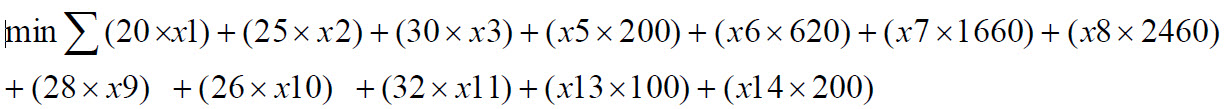# A Mixed-Integer Linear Programming (MILP) Problem for Generator Bids

## The Challenge

Two generators (G1 and G2) are competing to supply a 60MW load. The bidding information of the two generators is shown in Table 1 for G1 and in Table 2 for G2. The minimum and maximum capacities for G1 are 15MW and 65MW, respectively. The minimum and maximum capacities for G2 are 10MW and 80MW, respectively.

Assume each unit has a no-load cost. No-load cost for G1 is \$100. No-load cost for G2 is \$200.

### Bidding Tables

 Table 1. G1 Bidding Information Quantity (MW) Price (\$/MWh) 20 20 30 25 15 30

 Table 2. G2 Bidding Information Quantity (MW) Price (\$/MWh) 15 28 40 26 25 32

### Deliverables

Find the minimum cost to supply the load and the accepted quantities for G1 and G2. Formulate the problem using mixed-integer linear programming.

• Complete MILP formulation (variables, objective function, constraints, bounds)
• Optimal solutions (commitment of units, accepted quantities, cost to supply the load)

### Hints

• It’s possible that only one unit is needed to supply the load.
• If a unit is committed, a no-load cost will be incurred.
• Cost curve for G1 is convex when it’s committed. Cost curve for G2 is non-convex when it’s committed.

## Formulation

### Variables

There are 14 variables in my generator model problem: x1, x2, x3, x4, x5, x6, x7, x8,x9,x10,x11,x12,x13,x14. They are defined as given below:

 Variable Definition x1 G1 Accepted Quantity 20 MW Step x2 G1 Accepted Quantity 30 MW Step x3 G1 Accepted Quantity 15 MW Step x4 G1 = x1 + x2 + x3 or total MW to be accepted by G1 x5 G2 Indicator Variable x6 G2 Indicator Variable x7 G2 Indicator Variable x8 G2 Indicator Variable x9 G2 Variable x10 G2 Variable x11 G2 Variable x12 G2 = x5 + x6 + x7 + x8 + x9 + x10 + x11 or total MW to be accepted by G2 x13 Unit commitment status of G1 x14 Unit commitment status of G2

### Objective Function

The objective of the problem is to minimize cost of combined bids of both generators.The objective function is the sum of the unit price of the MW multiplied by the quantity to be accepted added to the other ranges and their respective products plus the no-load cost.

Note: In the MATLAB portion of the model, the x4 and x12 variables are set equal to 0 since it is not in the problem definition to minimize these.

### Constraints

The constraints of the problem are shown below:

### Bounds

The bounds of the model are defined in the problem statement and are shown below:

 Variable Lower Bound Upper Bound x1 0 20 x2 0 30 x3 0 15 x4 15 65 x5 0 1 x6 0 1 x7 0 1 x8 0 1 x9 0 Inf x10 0 Inf x11 0 Inf x12 10 80 x13 0 1 x14 0 1

### MATLAB Setup

See the setup of the formulation in the MATLAB code here.

## Solution

### Optimal Solution

The accepted quantity from each generator range is shown below and in the MATLAB results:

 G1 Accepted Quantities Information Quantity (MW) Price (\$/MWH) 20 20 30 25 10 30

 G2 Accepted Quantities Information Quantity (MW) Price (\$/MWH) 0 28 0 26 0 32

### Total Cost to Supply Load

Unit G2 is not committed, but G1 is committed with a total cost of:

20X20+30X25+10X30+100= \$1550

The total minimized cost to supply load is \$1550

## Code

You may download a copy of the project by visiting the code repo here.Kathleen has 15 yrs. experience analyzing business IT needs and engineering solutions in electric utilities, pharmaceutical, and internet industries with a variety of technologies. Kathleen's project experience has been diverse ranging from executing the business analysis “design phase” to hands-on implementation and testing of the IT solutions. She has also delivered technical training, user documentation, and developed new business processes for the solutions. Kathleen also has strengths in software development, programming, testing vendor applications, databases, creating business efficiency tools, and IT solutions with proficiency in technologies such as C# .NET applications, Visual Basic (VB.NET), SQL, Visual Studio, Oracle, XML, Microsoft applications, and more.